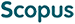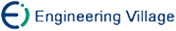Li Shunyong, Zhang Miaomiao, Cao Fuyuan. A MD fuzzy k-modes Algorithm for Clustering Categorical Matrix-Object Data[J]. Journal of Computer Research and Development, 2019, 56(6): 1325-1337. doi: 10.7544/issn1000-1239.2019.20180737
 Citation: Li Shunyong, Zhang Miaomiao, Cao Fuyuan. A MD fuzzy k-modes Algorithm for Clustering Categorical Matrix-Object Data[J]. Journal of Computer Research and Development, 2019, 56(6): 1325-1337. doi: 10.7544/issn1000-1239.2019.20180737# A MD fuzzy k-modes Algorithm for Clustering Categorical Matrix-Object Data• Traditional algorithms generally cluster single-valued attributed data. However, in practice, each attribute of the data object is described by more than one feature vector. For example, customers may purchase multiple products at the same time as they shop. An object described by multiple feature vectors is called a matrix object and such data are called matrix-object data. At present, the research work on clustering algorithms for categorical matrix- object data is relatively rare, and there are still many issues to be settled. In this paper, we propose a new matrix-object data fuzzy k-modes (MD fuzzy k-modes) algorithm that uses the fuzzy k-modes clustering process to cluster categorical matrix-object data. In the proposed algorithm, we introduce the fuzzy factor β with the concept of fuzzy set. The dissimilarity measure between two categorical matrix-objects is redefined, and the heuristic updating algorithm of the cluster centers is provided. Finally, the effectiveness of the MD fuzzy k-modes algorithm is verified on the five real-world data sets, and the relationship between fuzzy factor β and membership w is analyzed. Therefore, in the era of big data, clustering multiple records by using the MD fuzzy k-modes algorithm can make it easier to find customers’ spending habits and preferences, so as to make more targeted recommendation.
•/DownLoad:  Full-Size Img  PowerPoint